# How to Calculate Raster Statistics by Vector Polygon in QGIS

Statistics are important figures to perceive common conditions when working with data. Some statistics parameters like average, median, variance, standard deviation and many more are used to get a general overview  from data. Example, from 365 daily temperature data for a region within a year, it will be difficult to get a common sense from it. Therefore we use those statistics figures like minimum and maximum temperature, average temperature, variance, etc to get a quick overview.
As we know, a raster data contains pixel values that represent a specific variable. To get an overview about a raster value, we can use a raster statistics tool. But how to get raster statistics parameters based on polygon regions in a vector layer? I will discuss it in this tutorial and also plot the result in a graph as in figure 1.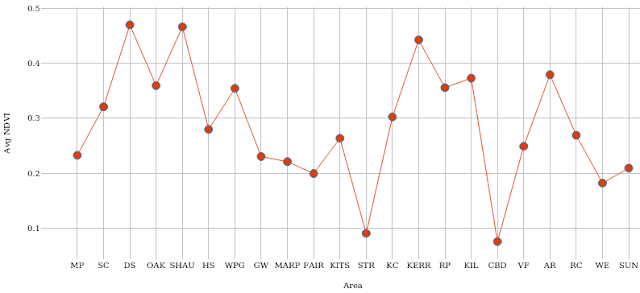Figure 1. Average NDVI for multiple local areas

### Raster Statistics by Vector Polygon Calculation

For this tutorial I used NDVI data and a local area boundary which contains 22 polygons from the city of Vancouver. The vector data can be downloaded from Vancouver Open Data Portal and the NDVI was calculated in Google Earth Engine (GEE) platform. If you're interested how to calculate spectral indices in GEE, check out this post:  How to Calculate Various Spectral Indices in Google Earth Engine Quickly

Now let's see how to calculate raster statistics by vector layer in QGIS.

Firstly add a raster and a vector layer that contains some polygons. In figure 2, can be seen I added a NDVI raster and a local boundary polygon as mentioned before.

Open the Processing Toolbox. In the search, type a keyword statistics. All tools that relate to this keyword will appear. Choose v.rast.stats from GRASS as in figure 3.

In the v.rast.stats tool window as in figure 4, select the vector polygon, raster data and specify a prefix for new generated statistics field(s).

Next in The methods to use option. There are 13 statistics parameters that will be computed. You can check/uncheck the option to calculate only certain parameters as in figure 5.

That's all what you need to play in the v.rast.stats tool window. After running the tool, a new vector layer with all computed statistics parameter fields will be added into the map. Figure 6 is the preview of  the attribute table from the new added layer. What can you observe? Is there something wrong?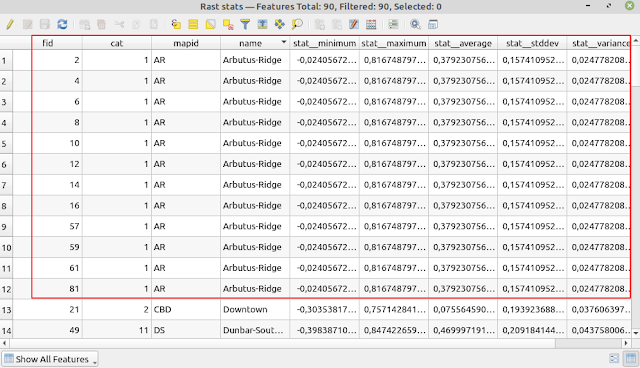Figure 6. Statistics parameters in attribute table

Yes, there is something wrong with it. Now there are 90 rows/polygons. It must be 22 polygons. Where are these new rows coming from? Honestly, I don't know. But fortunately, if you observe the value, they are all the same. To get rid of this, we can dissolve it based on a statistics parameter field. Moreover, I think it does not happen not for all cases, so it's better to check it before you're doing a dissolve operation.

### Dissolve Operation

To do a dissolve operation, from the top menu select Vector > Geoprocessing Tools > Dissolve... as shown in figure 7. Then from the Dissolve window select input vector and a field as in figure 8.

Now you should get a right number of polygon area as in the following figure.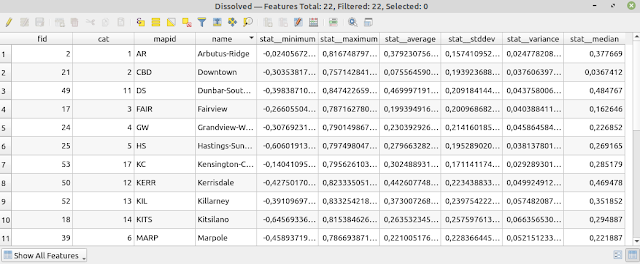Figure 9. Attribute table after dissolve

### Plotting The Graph

Congratulations! It's done. You've computed raster statistics based on vector polygon to get some parameters for each polygon as in figure 9. But we won't stop here. Because it's not easy to compare each figure in a table with many rows. So let's create a graph to make it easier to see the difference among them.

To create a graph in QGIS we use the Data Plotly plugin. It's not installed by default. Therefore you have to install it from Plugin > Manage and Install Plugins.. menu and make sure it's checked as in figure 10.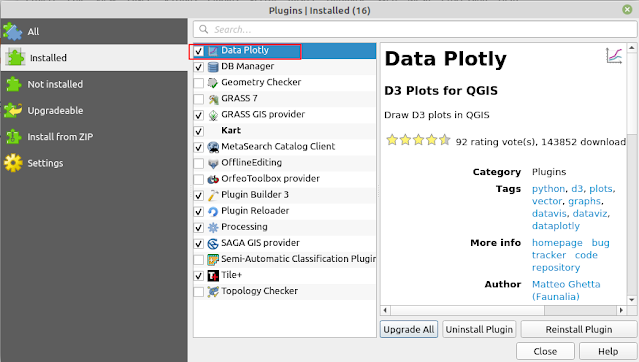Figure 10. Enabled Data Plotly Plugin

Open Data Plotly plugin by clicking the icon in the toolbar. The Data Plotly window should appear as in figure 11. From the window select a plot type. In this case I want to create a scatter plot. Then select the vector layer. Next define x and y fields. In the next section you can type a title for the legend. Because I want to make a line graph with points, so in the Marker type option I chose Points and Lines.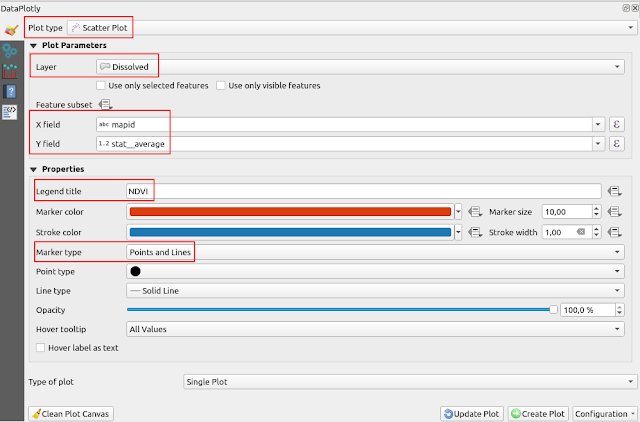Figure 11. Data Plotly window

Next, in the Layout options you can set a title for the graph and set x and y label as seen in figure 12.

After this step, you can create the graph by pushing Create Plot button in the bottom of the window and you should get a graph like figure 1.

That's all the tutorial on how to calculate raster statistics by vector layer in QGIS. We already discussed how to get raster statistics for each polygon in a vector layer using the v.rast.stats tool from GRASS and plot a graph to visualize it using the Data Plotly plugin. Thanks for reading!

In another post I discussed how to calculate summary statistics for selected features by location in QGIS. Check it out!